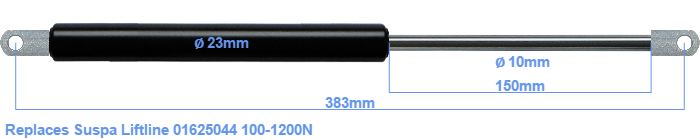# Replacement for Suspa Liftline 01625044 100-1200N

£34.19 (excl. VAT)

Replacement gas spring for the Suspa Liftline 01625044 100-1200 Newton. The eye has a thickness of 10mm and a hole diameter of 8.1mm. Brand: Hahn.

Please note:
• The thickness of the eye of this replacement is 10mm instead of 5mm.
• The diameter of the tube of this replacement gas spring is 23mm instead of 22mm.
•  Force Choose an option100 Newton120 Newton125 Newton140 Newton150 Newton160 Newton175 Newton180 Newton200 Newton220 Newton225 Newton240 Newton250 Newton260 Newton275 Newton280 Newton300 Newton320 Newton325 Newton340 Newton350 Newton360 Newton375 Newton380 Newton400 Newton420 Newton425 Newton440 Newton450 Newton460 Newton475 Newton480 Newton500 Newton520 Newton525 Newton540 Newton550 Newton560 Newton575 Newton580 Newton600 Newton620 Newton625 Newton640 Newton650 Newton660 Newton675 Newton680 Newton700 Newton720 Newton725 Newton740 Newton750 Newton760 Newton775 Newton780 Newton800 Newton820 Newton825 Newton840 Newton850 Newton860 Newton875 Newton880 Newton900 Newton920 Newton925 Newton940 Newton950 Newton960 Newton975 Newton980 Newton1000 Newton1020 Newton1025 Newton1040 Newton1050 Newton1060 Newton1075 Newton1080 Newton1100 Newton1120 Newton1125 Newton1140 Newton1150 Newton1160 Newton1175 Newton1180 Newton1200 NewtonClear
This gas spring is also known as 16-4 016 25044, 16-4-203-150-A23-B23.